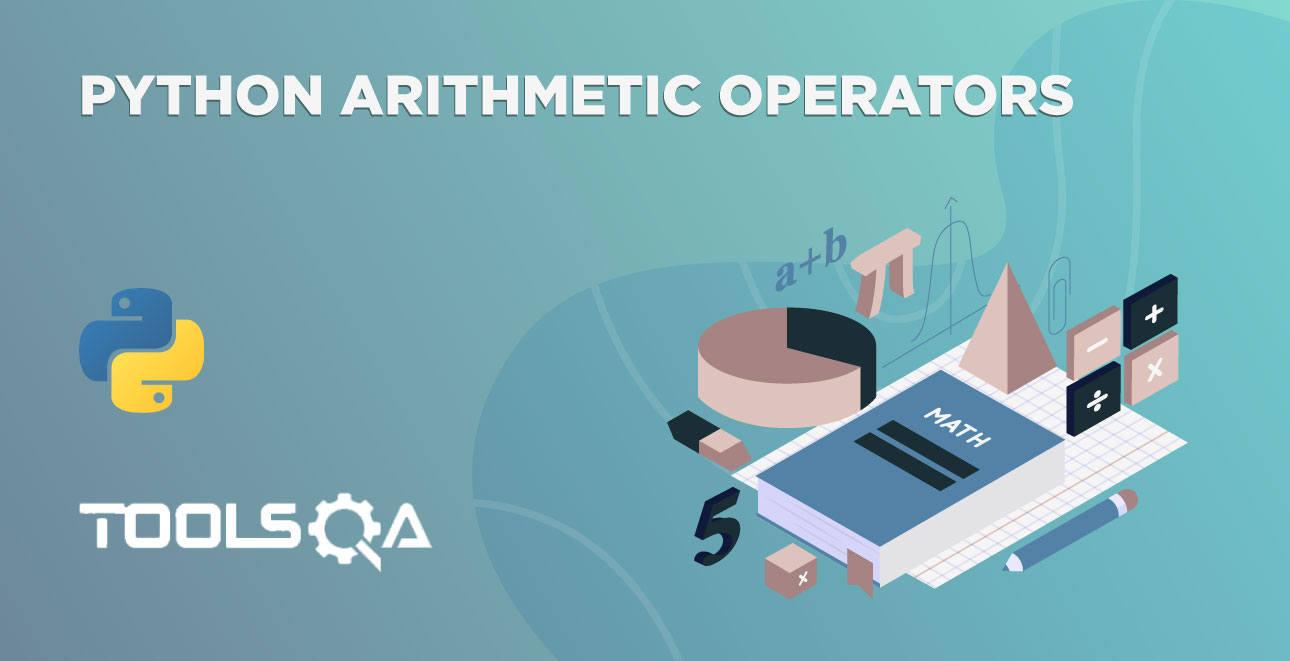We have learned about the basic arithmetic operators in the last tutorial. Additionally, we have used the python literals until the previous tutorial.  What if we want to operate with the result of its last operation? How to do it? The answer is simple. We have to store the result in the Python Variable. Subsequently, how to declare variables in Python? Hold the breath. We are going to see it in a bit. First, look at the table of contents of the tutorial.

• What is a Variable?
• Python Variable Syntax
• Python Variable Naming Conventions
• Python Keywords
• Python Variable Initialization

## What is a Variable in Programming?

We use Variables to store the values. Why do we have to save the values? The answer is simple- for future purposes. In other words, if you want to write a program that counts the number of even and odd numbers in a range, then you need to store the count to display it to the users.

Variables contain mainly two parts, one is a name, and another one is value. Moreover, Values may be of different data types.

### Python Variable Syntax

In Python, we use an operator called assignment operator(=) to assign or store the values into a variable. Additionally, an assignment operator is nothing but equals to (=) symbol. The left side of the assignment contains a name. In the same way, the right side of the assignment includes a value.

``````variable_name = value
``````

The variable_name is the name of the variable. Moreover, the value is the information or data to be stored in the variable. Subsequently, you will get to know the different types of data store in variables.

We have special rules for naming the variables in Python. We have to follow those rules to name the variables. Let's see how to call the variables in Python.

### Python Variable Naming Convention

Python has some rules to define the names of variables. We can't call the variables however we want. Therefore, we should follow the below rules while naming the variables.

• Firstly, Python allows using only alphabets, digits, and underscores (_) for the naming of variables.
• Secondly, Variable names must start with _ or alphabets. Moreover, the alphabets may be lowercase or uppercase.
• Thirdly, we can not use the keywords of  Python as a variable name. Keywords are reserved words of Python, which has unique functionality.
• Lastly, variables names in Python are case-sensitive, i.e., uppercase letters are different from lowercase letters. For example, ToolQA is different from toolsqa.

So, we now know the rules of naming variables in Python. Let's see some valid and invalid variables. Additionally, we will learn to detect the error of invalid variables in code.

• _3 is a valid variable name, as it follows all the above rules.
• ToolsQA is also a valid name.
• Moreover, 3ToolsQA is an invalid variable name, as it starts with a digit. We can't begin the variable with a figure.
• Tools@A is an invalid name, as it contains a unique character @.
• Similarly, Tools QA is an invalid name, as it contains a space.

Now you know the naming rules of a variable in Python. Answer whether False is a valid variable name or not.

If you are following the tutorials from the first, then you will know that False is one of the keywords in Python. Additionally, we can't use keywords as variable names. Hence, False is not a valid variable name in Python. Let's see other keywords.

### Python Keywords

Keywords are the reserved words in Python. Moreover, these keywords have different meanings for various purposes. See the list of keywords.

['False', 'None', 'True', 'and', 'as', 'assert', 'async', 'await', 'break', 'class', 'continue', 'def', 'del', 'elif', 'else', 'except', 'finally', 'for', 'from', 'global', 'if', 'import', 'in', 'is', 'lambda', 'nonlocal', 'not', 'or', 'pass', 'raise', 'return', 'try', 'while', 'with', 'yield']

We can't use the above keywords to name variables in our code. But, we can use them by changing the case of any letter in the keyword. For example,

We can't name our variable as follows, as print is a keyword in Python.

``````print
``````

But, we can name our variable by changing the case of any letter in the keyword print.

``````Print
``````

Consequently, the above variable name is a valid one. We will learn about the keywords in the corresponding tutorials.

We have learned the rules of naming the variables in Python. Try to name the variables based on the situation and context of the program. Additionally, it will be easy to read the code if you use the right names in the proper context. For example, if you are calculating the area of a triangle, it's better to give a variable name as an area instead of declaring it randomly as xyz.

### Python Variable Initialization

What is Initialization? Assigning an initial value to a variable is generally known as initialization. In other words, you can think of it as giving importance to the variable for the first time.

Python is a dynamically typed language that has some advantages over other programming languages.

In other programming languages like C, Java, C++, etc. we have to define a variable with a data type. Moreover, if you want to store an integer value in a variable, you have to declare it as int variable_name. Defining variables creates a memory for the variable. Additionally, the variables with int data type only store integers. They can't store other data types like float, string, char, etc.

But in Python, we don't have to declare the data type of variables. We can store any data in a variable. In other words, we will directly initialize the variable with any value. Python variables can store any data—one of the most liked features of Python.

To create a variable in Python, we will use the assignment operator (=), as discussed at the starting of this tutorial. That is to say, name the variable and assign the value to it. That's it. You have created a variable.

``````toolsqa = 2019
``````

In the above code, we have created a variable called ToolsQA and assign a value 2019. Check it by printing it to the output console.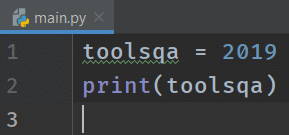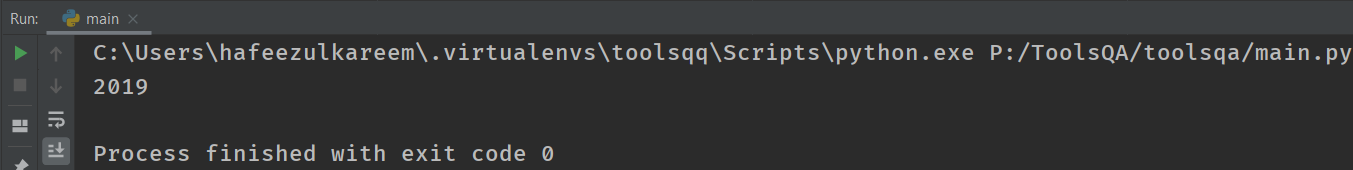Let's see some more examples and different situations.

``````# variables
toolsqa = 2020
print(toolsqa)
``````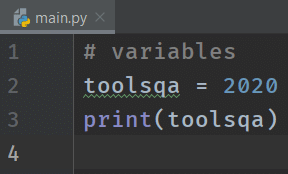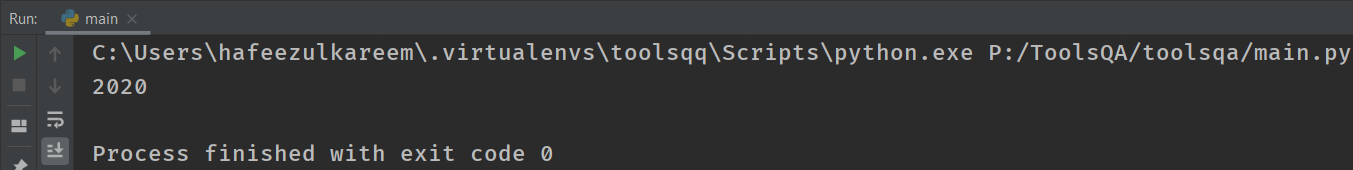We can use any number of variables in the code. There is no limit to that. Additionally, we can't use variables that the program does not create.

The following example gives us an error.

``````# variables
toolsqa = 2020
print(Toolsqa)
``````

We don't have the variable Toolsqa in the code. And remember toolsqa and Toolsqa are two different variables as Python is case-sensitive.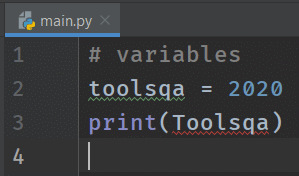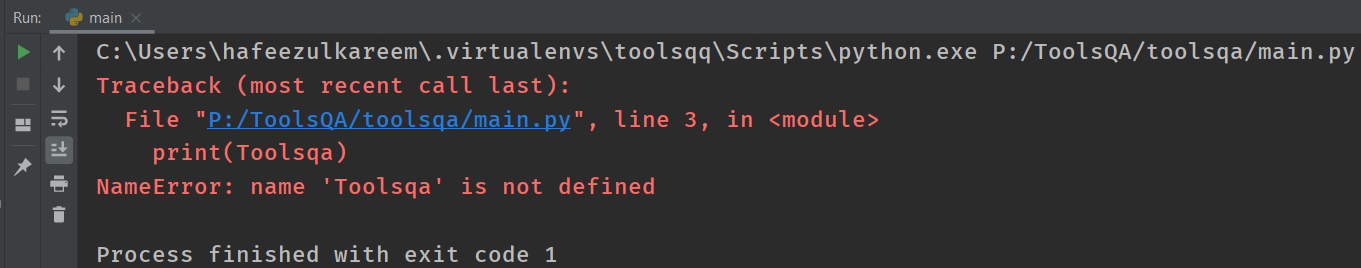We got the NameError: name 'Toolsqa' is not defined error, as discussed earlier. The program does not create the variable with the name Toolsqa.

What happens when we use invalid names in the code? We will get an error. Let's see what it is.

``````# variables
toolsq@ = 2020
print(toolsq@)
``````

Is the variable toolsq@ invalid? Yeah! It is. We have a distinctive character @ in the variables name, which is not allowed in Python. Run the code and see the error in IDE.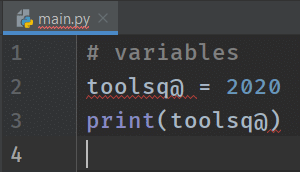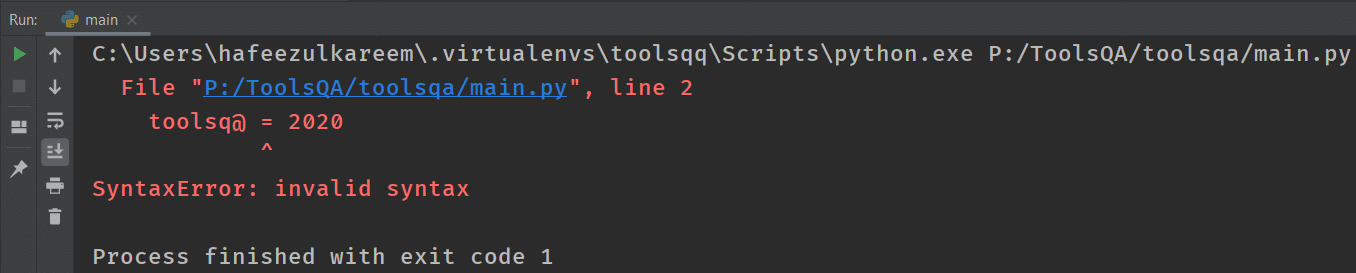The interpreter shows the error as we expected. We got SyntaxError, which is the most common type of error in the programming languages. Invalid syntax means that the interpreter can't understand the code you have written. It's like talking in English to a person who doesn't know the English. Python is not allowing us to use the special characters in variables names.

Try the following variable names in your code.

• _toolsqa
• tools qa
• 2020toolsqa
• toolsqa2020

Let's say we have a program in which we have to update a variable's value in different situations. How to update or assign a new value to the existing variable? It's just like creating a variable. For example,

``````# variables
toolsqa = 2019
print(toolsqa)
toolsqa = 2020
print(toolsqa)
``````
• We have created the variable toolsqa with value 2019.
• Next, we assigned a new value of the variable toolsqa.We can have variables, expressions, or literals on the right side of the variable assignment. Let's see one more example where we update the value of a variable with an expression.

``````# variables
toolsqa = 2019
print(toolsqa)
toolsqa = toolsqa + 1
print(toolsqa)
``````
• The first line of the code creates variable toolsqa with value 2019. You can read it as assign 1 to toolsqa.
• In the third line, we try to update the value of toolsqa with the value of the same variable with the addition of 1. Here, we are incrementing the value of toolsqa by 1.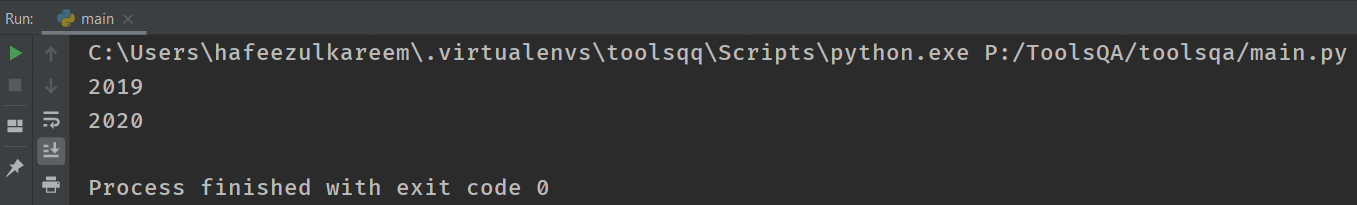In the previous code,

Some more examples related to incrementing the value.

``````# variables
toolsqa = 2019
print(toolsqa)
toolsqa = toolsqa + 5
print(toolsqa)
``````

We are incrementing the value of toolsqa by 5. Guess the output. We can also assign a complex expression to the variable instead of direct value. Assign one expression to the variable that we have seen in the last tutorial. Take the following example as a reference.

``````# variables
result = (5 - 2) - 2 * 5
print(result)
``````

Instead of direct value, we have assigned an expression to the variable result. Python first evaluates the expression and then assigns the result to the respective variable. Evaluate the expression and check it in the IDE.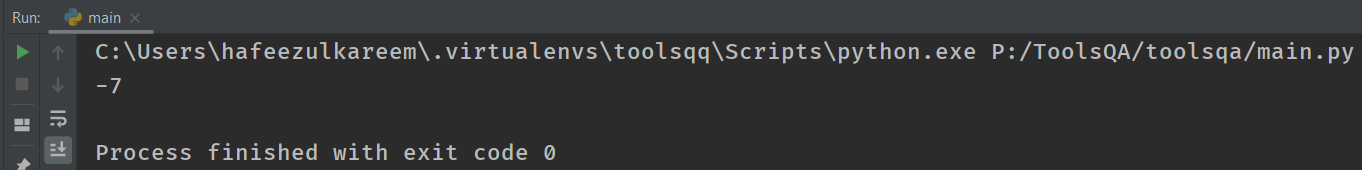### Key Takeaways

• Variables make programmers' life easy by saving the data in them.
• We can't use Python keywords as variable names.
• We can use builtin-in Python functions as variable names, but we don't recommend it.
• Python is a case-sensitive language, i.e., the variable Toolsqa is different from toolsqa.
• We are not allowed to use special characters or spaces in variable naming except underscore (_). E.g., Tools_QA is permitted, but Tools QA or Tools@QA is not allowed
• We should use an alphabet or underscore (_) to start a variable name.
• One can initialize a variable with a value using the assignment operator (=)—for example, a = 5.
• We can update the value of the variable by reassigning the value to the same variable. For example, a = 5; a = 4;.

## Python Variable - Practice Yourself

We have learned about the expressions in the last tutorial and variables in this tutorial. Combining both, let's write the expression for the formula (a + b)2 in Python.

(a + b) ** 2

Now, write the expression for the expansion of the above formula, i.e., a2 + b2 + 2ab. After writing the expression, check it with values 2 and 3 for a and b, respectively. You can take the following code as a reference.

``````#Problem 1
a = 2
b = 3
expression = _____
print(expression)
``````
``````#Problem 2
_1 = 1
_2 = 5
_3 = 5
_4 = 1
point_distance = ((_1 - _3) ** 2 + (_2 - _3) ** 2) ** 0.5
print("Distance ", point_distance)
``````
``````#Problem 3
company_name = "ToolsQA"
year = 2020
print(company_name, year)
``````
``````#Problem 4
None = "No Value"
print(None)
``````
``````#Problem 5
bool = "True"
print(bool)
``````
``````#Problem 6
side = 4
area_of_square = side * side
print(area_of_square)
``````
``````#Problem 7
# Follow the below steps and write the program
# 1. Initialize three variables a, b, and c with some random values
# 2. Print them and seperate with a star
# 3. Multiply three variables and assign the result to a new variable called product
# 4. Print the result as "Product: " and product
``````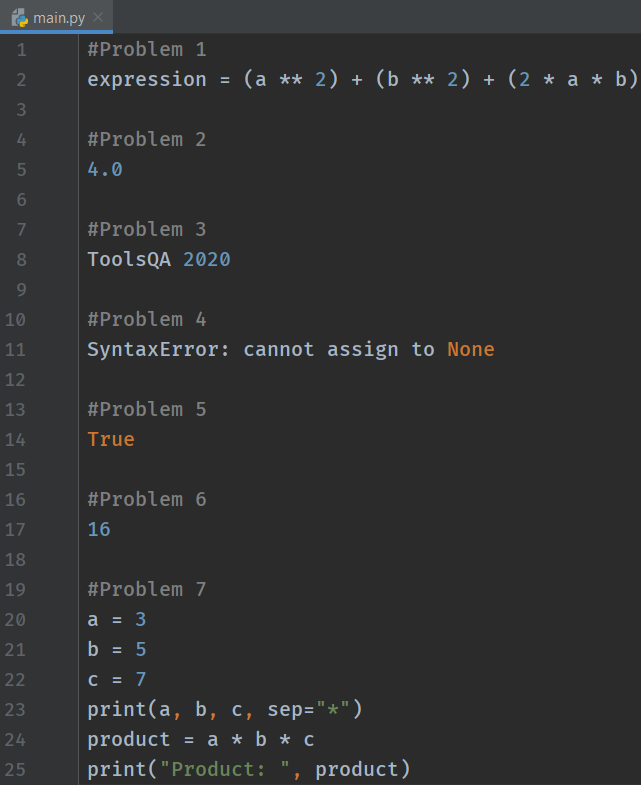### What's Next?

To conclude, we have learned about variables and keywords in this tutorial. Make sure that you learned how to name the variables. You know the variable naming rules and keywords. This knowledge is high enough to call variables without any errors.

We have seen the assignment operator in this tutorial. Python has some special operators which reduce the length of the variable updating statement. They are called shortcut operators. We will explore it in the next tutorial.

Happy Coding :)